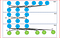# Deep learning — Deep Boltzmann Machine (DBM)

In this post we will discuss what is deep boltzmann machine, difference and similarity between DBN and DBM, how we train DBM using greedy layer wise training and then fine tuning it.

Deep Boltzmann was proposed by :

Salakhutdinov, Ruslan & Larochelle, Hugo. (2010). Efficient Learning of Deep Boltzmann Machines.. Journal of Machine Learning Research — Proceedings Track. 9. 693–700.

• Unsupervised, probabilistic, generative model with entirely undirected connections between different layers
• Contains visible units and multiple layers of hidden units
• Like RBM, no intralayer connection exists in DBM. Connections exists only between units of the neighboring layers
• Network of symmetrically connected stochastic binary units
• DBM can be organized as bipartite graph with odd layers on one side and even layers on one side
• Units within the layers are independent of each other but are dependent on neighboring layers
• Learning is made efficient by layer by layer pre training — Greedy layer wise pre training slightly different than done in DBM
• After learning the binary features in each layer, DBM is fine tuned by back propagation.

Sounds similar to DBN so what is the difference between Deep Belief networks(DBN) and Deep Boltzmann Machine(DBM)?

Let’s talk first about similarity between DBN and DBM and then difference between DBN and DBM

• Both DBN and DBM are unsupervised, probabilistic, generative, graphical model consisting of stacked layers of RBM.
• DBN and DBM both are used to identify latent feature present in the data.
• Both DBN and DBM performs inference and parameter learning efficiently using greedy layer–wise training.
• Both DBN and DBM apply discriminative fine tuning after greedy layer wise pre training.
• Both DBN and DBM use a large set of unlabeled data for pre training in an unsupervised manner to find good set of parameter for the model and then apply discriminative fine tuning on a small labelled dataset.
• Deep Belief Network(DBN) have top two layers with undirected connections and lower layers have directed connections
• Deep Boltzmann Machine(DBM) have entirely undirected connections.
• Approximate inference procedure for DBM uses a top-down feedback in addition to the usual bottom-up pass, allowing Deep Boltzmann Machines to better incorporate uncertainty about ambiguous inputs.
• A disadvantage of DBN is the approximate inference based on mean field approach is slower compared to a single bottom-up pass as in Deep Belief Networks. Mean field inference needs to be performed for every new test input.

Explaining mean field or variational approximation intuitively here

Computing posterior distribution is known as an inference problem.

We have a data distribution P(x) and computing posterior distribution is often intractable.

We can approximate intractable inference with simpler tractable inference by introducing a distribution Q(x) which is the best approximation of P(x).

Q(x) becomes the mean field approximation where variables in Q distribution is independent of variable x.

Our goal is to minimize KL divergence between the approximate distribution and the actual distribution

Boltzmann machine uses randomly initialized Markov chains to approximate the gradient of the likelihood function which is too slow to be practical.

DBM uses greedy layer by layer pre training to speed up learning the weights. It relies on learning stacks of Restricted Boltzmann Machine with a small modification using contrastive divergence.

The key intuition for greedy layer wise training for DBM is that we double the input for the lower-level RBM and the top level RBM.

Lower level RBM inputs are doubled to compensate for the lack of top-down input into first hidden layer.Similarly for top-level RBM, we double the hidden units to compensate for the lack of bottom-up input.

For the intermediate layers, the RBM weights are simply doubled.Double inputs for the input and the top level hidden layer. Double the weights for the intermediate layers

The 3 RBM’s are then combined to form a single model.

Greedily pretraining the weights of a DBM initializes the weights to reasonable values helping subsequent joint learning of all layers.

This is expensive compared to a single bottom up inference used in DBN. In order to learn using large dataset we need to accelerate inference in a DBM.

In order to accelerate inference of DBM, we use a set of recognition weights, which are initialized to the weights found by the greedy pre training.

We take an input vector and apply the recognition weights to reconstruct the input v of fully factorized approximation posterior distribution.

Each layer of hidden units is activated in a single deterministic bottom-up pass as shown in figure below.

We double the weights of the recognition model at each layer to compensate for the lack of top-down feedback. However we do not double the top layer as it does not have a top-down input.

We apply K iterations of mean-field to obtain the mean-field parameters that will be used in the training update for DBM’s.

Finally we update the recognition weights for an initial guess of the input ν close to the result µ. µ is the result of the mean field inference which is ur target

By updating the recognition weights we want to minimize the KL divergence between the mean-field posterior (h|v; µ) and the recognition model.Objective is to minimize KL divergence between mean field posterior and factorial posterior of the recognition model

To perform classification, we need a separate multi layer perceptrons(MLP) on top of the hidden features extracted from greedy layer pre training just as fine tuning is performed in DBN

http://proceedings.mlr.press/v5/salakhutdinov09a/salakhutdinov09a.pdf

http://proceedings.mlr.press/v9/salakhutdinov10a/salakhutdinov10a.pdf

https://cedar.buffalo.edu/~srihari/CSE676/20.4-DeepBoltzmann.pdf

https://www.researchgate.net/publication/220320264_Efficient_Learning_of_Deep_Boltzmann_Machines

Written by